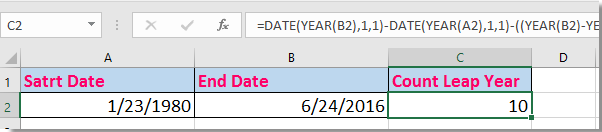## How to count number of leap years between two dates in Excel?

In Excel, to count the number of years between two given dates may be easy for most of users, but, can you only count the number of leap years also called intercalary year between two dates? Here I introduce a formula can help you to quickly calculate the number of leap years between a date range in Excel.

Count leap years in a date range with formula

####Count leap years in a date range with formula

To count leap years between two dates, you just need to do as this:

Select a blank cell that you will place the counted result at, C2 for instance, and enter this formula
=DATE(YEAR(B2),1,1)-DATE(YEAR(A2),1,1)-((YEAR(B2)-YEAR(A2))*365)+AND(MONTH(DATE(YEAR(A2),2,29))=2,MONTH(DATE(YEAR(B2),2,29))=2)*1
then press Enter key to get the result. See screenshot:Tip: In the formula, A2 is the start date and B2 is the end date, you can change them as you need.

### Best Office Productivity Tools

Supercharge Your Spreadsheets： Experience Efficiency Like Never Before with Kutools for Excel

 Popular Features: Find/Highlight/Identify Duplicates   |  Delete Blank Rows   |  Combine Columns or Cells without Losing Data   |   Round without Formula ... Super Lookup: Multiple Criteria VLookup  |   Multiple Value VLookup  |   VLookup Across Multiple Sheets   |   Fuzzy Lookup .... Advanced Drop-down List: Quickly Create Drop Down List   |  Dependent Drop Down List   |  Multi-select Drop Down List .... Column Manager: Add a Specific Number of Columns   |   Move Columns   |   Unhide Columns   |   Compare Columns to Select Same & Different Cells ... Featured Features: Grid Focus   |  Design View   |   Big Formula Bar   |  Workbook & Sheet Manager   |  Resource Library (Auto Text)   |  Date Picker   |  Combine Worksheets   |  Encrypt/Decrypt Cells   |  Send Emails by List   |  Super Filter   |   Special Filter (filter bold/italic/strikethrough...) ... Top 15 Toolset:  12 Text Tools (Add Text, Remove Characters, ...)   |   50+ Chart Types (Gantt Chart, ...)   |   40+ Practical Formulas (Calculate age based on birthday, ...)   |   19 Insertion Tools (Insert QR Code, Insert Picture from Path, ...)   |   12 Conversion Tools (Numbers to Words, Currency Conversion, ...)   |   7 Merge & Split Tools (Advanced Combine Rows, Split Cells, ...)   |   Many More...

Kutools for Excel boasts over 300 features, ensuring that what you need is just a click away...

Supports Office/Excel 2007-2021 & newer, including 365   |   Available in 44 languages   |   Enjoy a full-featured 30-day free trial.#### Office Tab Brings Tabbed interface to Office, and Make Your Work Much Easier

• Enable tabbed editing and reading in Word, Excel, PowerPoint, Publisher, Access, Visio and Project.
• Open and create multiple documents in new tabs of the same window, rather than in new windows.
• Increases your productivity by 50%, and reduces hundreds of mouse clicks for you every day!No ratings yet. Be the first to rate!
This comment was minimized by the moderator on the site
A more useful function would be to calculate the number of times Feb 29 occurs between 2 dates.
This comment was minimized by the moderator on the site
Tentei a fórmula acima, mas ainda gerava erro no numero de anos bissextos, então fiz uma função em VBA que faz a verificação ano a ano no intervalo.
Após incluir esta macro abaixo, para utilizar use a função =ContBissexto(A1;B1), o resultado é a quantidade de anos bissextos no período: Espero ter ajudado:

Function ContBissexto(Ini As Date, Fim As Date) As Integer
On Error Resume Next

Dim AnoIni As Integer
Dim AnoFim As Integer
Dim contB As Integer
contB = 0
'DEFINE O ANO DE INICIO DA CONTAGEM DO PERÍODO BISEXTO
If Ini <= DateSerial(Year(Ini), 3, 1) - 1 Then
AnoIni = Year(Ini)
Else
AnoIni = Year(Ini) + 1
End If
'DEFINE O ANO DE FIM DA CONTAGEM DO PERÍODO BISEXTO
If Fim > DateSerial(Year(Fim), 2, 28) Then
AnoFim = Year(Fim)
Else
AnoIni = Year(Fim) - 1
End If

For i = AnoIni To AnoFim
If Day(DateSerial(i, 3, 1) - 1) = 29 Then contB = contB + 1
Next

ContBissexto = contB

End Function
This comment was minimized by the moderator on the site
A contagem gerava erro no numero de anos bissextos, então fiz uma função em VBA que faz a verificação ano a ano no intervalo.
Após incluir esta macro abaixo, para utilizar use a função =ContBissexto(A1;B1), o resultado é a quantidade de anos bissextos no período:

Function ContBissexto(Ini As Date, Fim As Date) As Integer
On Error Resume Next

Dim AnoIni As Integer
Dim AnoFim As Integer
Dim contB As Integer
contB = 0
'DEFINE O ANO DE INICIO DA CONTAGEM DO PERÍODO BISSEXTO
If Ini <= DateSerial(Year(Ini), 3, 1) - 1 Then
AnoIni = Year(Ini)
Else
AnoIni = Year(Ini) + 1
End If
'DEFINE O ANO DE FIM DA CONTAGEM DO PERÍODO BISSEXTO
If Fim > DateSerial(Year(Fim), 2, 28) Then
AnoFim = Year(Fim)
Else
AnoIni = Year(Fim) - 1
End If

For i = AnoIni To AnoFim
If Day(DateSerial(i, 3, 1) - 1) = 29 Then contB = contB + 1
Next

ContBissexto = contB

End Function
This comment was minimized by the moderator on the site
Oi amigo,

Excelente fórmula!

Trabalhei com ela e percebi que há uma divergência quando o primeiro e/ou o segundo intervalo são anos bissextos. Tomei a liberdade de corrigir (em português):

=DATA(ANO(B2);1;1)-DATA(ANO(A2);1;1)-((ANO(B2)-ANO(A2))*365)+E(ANO(A2)=ANO(B2);MÊS(DATA(ANO(A2);2;29))=2;A2<=DATA(ANO(A2);2;29);B2>=DATA(ANO(B2);2;29))+E(ANO(A2)
There are no comments posted here yet